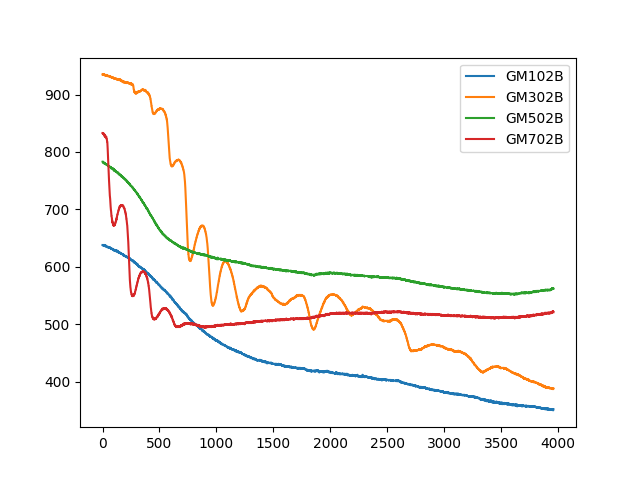# Grove Multichannel Gas Sensor V2: strange measurements from garlic

Hi everyone,

After playing around with my newly acquired gas sensor, I decided to measure the smell of a garlic:

.
The code to do the measurements in arduino is as follows:

``````
#include <Multichannel_Gas_GMXXX.h>

#ifdef SOFTWAREWIRE
#include <SoftwareWire.h>
SoftwareWire myWire(3, 2);
GAS_GMXXX<SoftwareWire> gas;
#else
#include <Wire.h>
GAS_GMXXX<TwoWire> gas;
#endif

static uint8_t recv_cmd = {};

void setup() {
Serial.begin(9600);
gas.begin(Wire, 0x08);
}

void loop() {
uint8_t len = 0;
uint8_t i;
uint32_t val = 0;
float val_vol = 0.0;

char gm102b_sensor[] = "GM102B\n";
char gm302b_sensor[] = "GM302B\n";
char gm502b_sensor[] = "GM502B\n";
char gm702b_sensor[] = "GM702B\n";

val = gas.getGM102B();
val_vol = gas.calcVol(val);
// Serial.print("GM102B: "); Serial.print(val); Serial.print("  =  ");
// Serial.print(val_vol); Serial.println("V");
Serial.write(gm102b_sensor, sizeof(gm102b_sensor));
Serial.write('u');
Serial.write((uint8_t*)&val, sizeof(val));
Serial.write('f');
Serial.write((uint8_t*)&val_vol, sizeof(val_vol));

val = gas.getGM302B();
val_vol = gas.calcVol(val);
// Serial.print("GM302B: "); Serial.print(val); Serial.print("  =  ");
// Serial.print(val_vol); Serial.println("V");
Serial.write(gm302b_sensor, sizeof(gm302b_sensor));
Serial.write('u');
Serial.write((uint8_t*)&val, sizeof(val));
Serial.write('f');
Serial.write((uint8_t*)&val_vol, sizeof(val_vol));

val = gas.getGM502B();
val_vol = gas.calcVol(val);
// Serial.print("GM502B: "); Serial.print(val); Serial.print("  =  ");
// Serial.print(val_vol); Serial.println("V");
Serial.write(gm502b_sensor, sizeof(gm502b_sensor));
Serial.write('u');
Serial.write((uint8_t*)&val, sizeof(val));
Serial.write('f');
Serial.write((uint8_t*)&val_vol, sizeof(val_vol));

val = gas.getGM702B();
val_vol = gas.calcVol(val);
// Serial.print("GM702B: "); Serial.print(val); Serial.print("  =  ");
// Serial.print(val_vol); Serial.println("V");
Serial.write(gm702b_sensor, sizeof(gm702b_sensor));
Serial.write('u');
Serial.write((uint8_t*)&val, sizeof(val));
Serial.write('f');
Serial.write((uint8_t*)&val_vol, sizeof(val_vol));

delay(10000);
}
``````

The data I obtained from the garlic (and environment) was as follows:, where for instance a datapoint for GM102B corresponds to `val = gas.getGM102B();`.

My background is data science / computer vision. From camera’s, my experience is that we often have constant measurements (same images), but these measurements are currently not very constant. From what I read, one reason could be that there is sensor drift, which we should calibrate for. However, I’m unsure whether sensor drift is the reason for these downwards sinusoidal curves for GM702B and especially GM302B.

I’m still very new in the sensor world, but am eager to learn what is going on in my case and how to mitigate issues like these when dealing with sensors. Can somebody explain what went wrong and how to mitigate (if possible) the issues that I’m seeing here?

Looking forwards to your replyDoes anyone know what could be the issue? Thanks!